# Thermochemical CalculationsPage 1

#### WATCH ALL SLIDES

Slide 1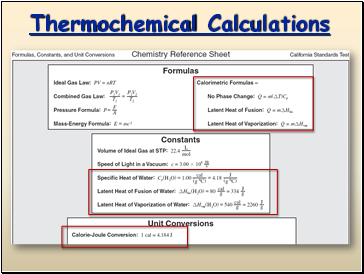## Thermochemical Calculations

Slide 2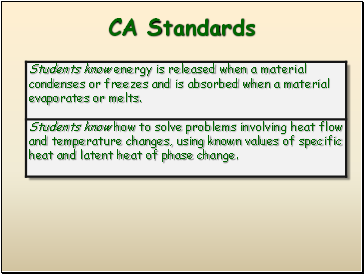CA Standards

Slide 3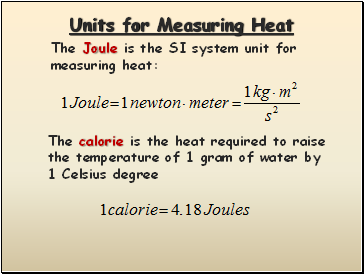## Units for Measuring Heat

The Joule is the SI system unit for measuring heat:

The calorie is the heat required to raise the temperature of 1 gram of water by 1 Celsius degree

Slide 4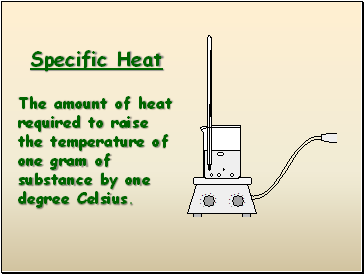## Specific Heat

The amount of heat required to raise the temperature of one gram of substance by one degree Celsius.

Slide 5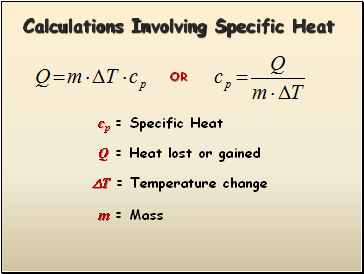## Calculations Involving Specific Heat

cp = Specific Heat

Q = Heat lost or gained

T = Temperature change

OR

m = Mass

Slide 6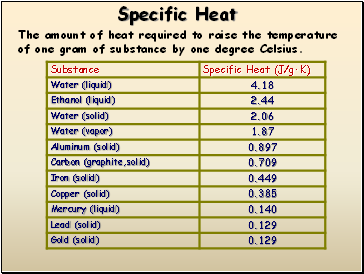## Specific Heat

The amount of heat required to raise the temperature of one gram of substance by one degree Celsius.

Slide 7## Latent Heat of Phase Change

Molar Heat of Fusion

The energy that must be absorbed in order to convert one mole of solid to liquid at its melting point.

The energy that must be removed in order to convert one mole of liquid to solid at its freezing point.

Molar Heat of Solidification

Slide 8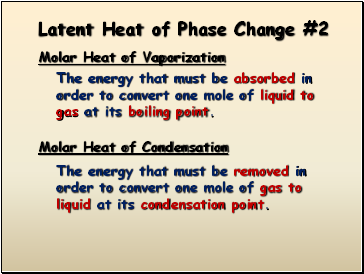Latent Heat of Phase Change #2

Molar Heat of Vaporization

The energy that must be absorbed in order to convert one mole of liquid to gas at its boiling point.

The energy that must be removed in order to convert one mole of gas to liquid at its condensation point.

Molar Heat of Condensation

Slide 9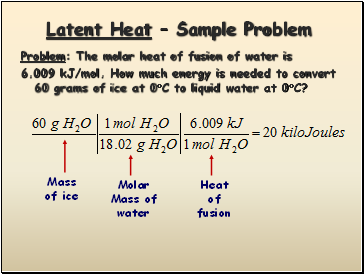## Latent Heat – Sample Problem

Problem: The molar heat of fusion of water is

6.009 kJ/mol. How much energy is needed to convert 60 grams of ice at 0C to liquid water at 0C?

Mass

of ice

Molar

Mass of

water

Heat

of

fusion

Slide 10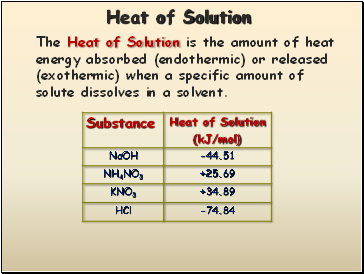## Heat of Solution

The Heat of Solution is the amount of heat energy absorbed (endothermic) or released (exothermic) when a specific amount of solute dissolves in a solvent.